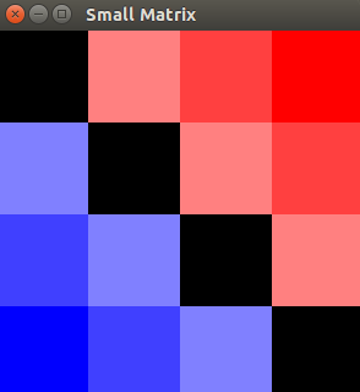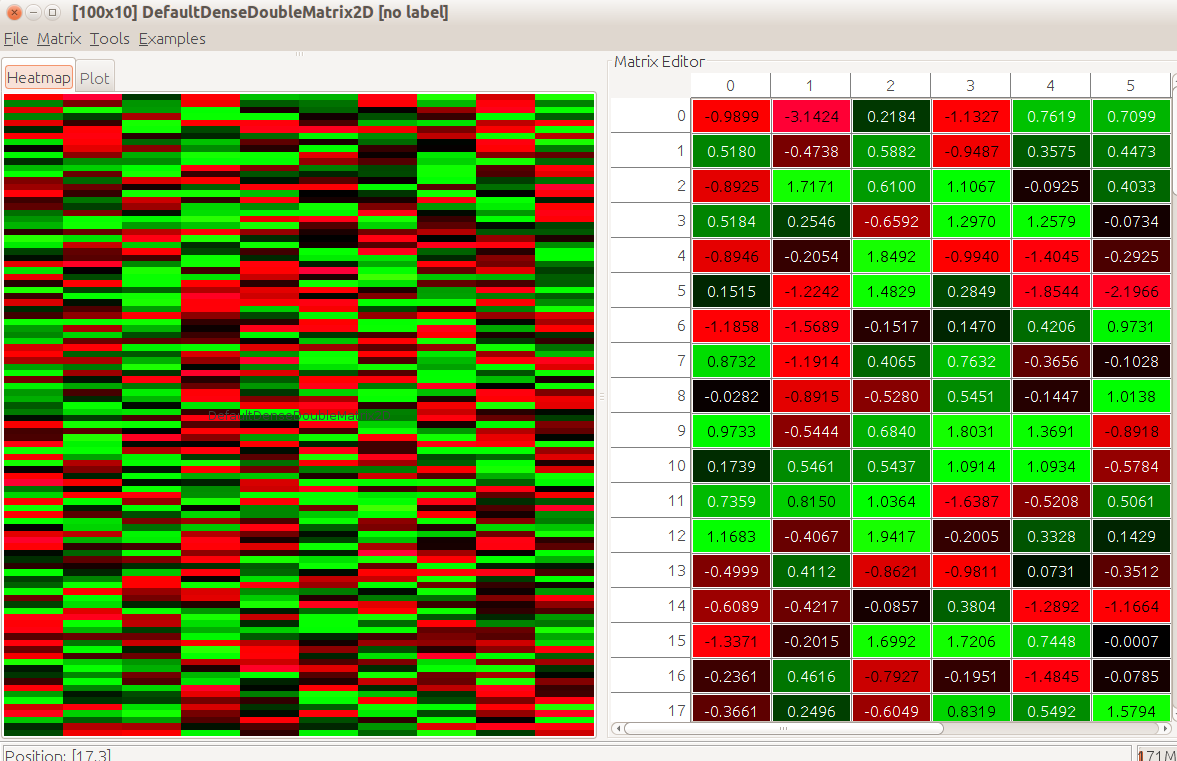# DMelt:Numeric/1 Linear Algebra

Limitted access. First login to DataMelt if you are a full DataMelt member. Then login to HandWiki as a user.

## Linear Algebra

Linear algebra is the branch of mathematics concerning linear equations. Read about linear algebra. The DataMelt contains many high-performance Java packages for linear algebra and matrix operations, such as

## Vectors

For manipulations with vectors, use the following "core" classes with useful static methods:

You can also use the Python list as a container to hold and manipulate with 1D data structures, such as P0I and P0D arrays. In addition, DataMelt supports 3rd-party vectors and their methods:

Below we show how to use static methods by mixing Python lists with the static methods of theArrayMath Java class:

from jhplot.math.ArrayMath import *
a=[-1,-2,3,4,5,-6,7,10] # make a Python list
print a
b=invert(a)             # invert it
print b.tolist()
c=scalarMultiply(10, b) # scalar multiply by 10
print c.tolist()

print mean(a)
print sumSquares(a)     # sums the squares

This code generates the following output:

[-1, -2, 3, 4, 5, -6, 7, 10]
[10, 7, -6, 5, 4, 3, -2, -1]
[100.0, 70.0, -60.0, 50.0, 40.0, 30.0, -20.0, -10.0]
2.5
240

## Matrices

A large choice matrix manipulation provided by DataMelt is shown below. The core DataMelt packages include the following implementation:

Please look at DataMelt API to see all Java implementations of matricies from different packages. Here are examples:

For matrix calculations, consider the packageLinearAlgebra. A simple example below can illustrate how to get started:

from jhplot.math.LinearAlgebra import *
array = [[1.,2.,3],[4.,5.,6.],[7.,8.,10.]]
inverse=inverse(array)  # calculate inverse matrix
print inverse
print trace(array)      # calculate trace

While working with NxM matrices, consider another important libraryDoubleArray which helps to manipulate with double arrays. For example, this class has toString() method to print double arrays in a convenient format. Consider this example:

from jhplot.math.LinearAlgebra import *
from jhplot.math.DoubleArray import *
print dir() # list all imported methods

array = [[1.,2.,3],[4.,5.,6.],[7.,8.,10.]]
inverse=inverse(array)
print toString("%7.3f", inverse.tolist()) # print the matrix

The above script prints all the methods for matrix manipulation and the inverse matrix itself:

-0.667  -1.333   1.000
-0.667   3.667  -2.000
1.000  -2.000   1.000

## Scripting using Jama

The JavaJama package allows allows matrix creation and manipulation. Below is a simple example of how to call Jama package to create a matrix to perform some manipulations.

from Jama import *
array = [[1.,2.,3],[4.,5.,6.],[7.,8.,10.]]
a = Matrix(array)
b = Matrix.random(3,1)
x = a.solve(b)
Residual = a.times(x).minus(b);
rnorm = Residual.normInf();

To print a matrix, one can make a simple function that converts a matrix to a string:

from Jama import *
def toString(a):
s=""
for i in range(a.getRowDimension()):
for j in range(a.getColumnDimension()):
s=s+str(a.get(i,j))+"    "
s=s+ "\n"
return s

print toString(a) # print "a" (must be Matrix object)

Here is a summary of Jama capability. Please readJama API for detailed description.

## Linear Algebra with Apache Math

For matrix manipulation, one can also use Apache Math Common Linear Algebra package: Look at theApache API for linear algebra. Below we show a simple example of how to create and manipulate with matrices:

from org.apache.commons.math3.linear  import *

# Create a real matrix with two rows and three columns
matrixData = [[1,2,3], [2,5,3]]
m=Array2DRowRealMatrix(matrixData)

# One more with three rows, two columns
matrixData2 = [[1,2], [2,5], [1, 7]]
n=Array2DRowRealMatrix(matrixData2)

# Now multiply m by n
p = m.multiply(n);
print p.getRowDimension()    # print 2
print p.getColumnDimension() # print 2

# Invert p, using LU decomposition
inverse =LUDecompositionImpl(p).getSolver().getInverse();

## Dense and sparse matrices

la4j package provides a simple API to handle sparse and dense matrices. According to the La4j authors, the package has Linear systems solving (Gaussian, Jacobi, Zeidel, Square Root, Sweep and other), Matrices decomposition (Eigenvalues/Eigenvectors, SVD, QR, LU, Cholesky and other), and useful I/O (CSV and MatrixMarket format).

Let us consider how we define such matrices in this package:

Let us show how to perform manipulations with such matrices. In the example shown below, we multiply matrices and then perform a transformation of matrices using an arbitrary function:

## Dense matrices in EJML

The EJML package provides 2 types of matrices:

EJML library provides the following operations:

• Basic Matrix Operators (addition, multiplication ... )
• Matrix Manipulation (extract, insert, combine... )
• Linear Solvers (linear, least squares, incremental... )
• Matrix Decompositions (LU, QR, Cholesky, SVD, Eigenvalue ...)
• Matrix Features (rank, symmetric, definitiveness ... )
• Creating random Matrices (covariance, orthogonal, symmetric ... )
• Different Internal Formats (row-major, block)
• Unit Testing
• Saving matrices into CSV files

Let us give a simple example using Jython: We create a few matrices and perform some algebra (multiplication, inverse etc). We also computes the eigen value decomposition and will print the answer:

You can test various features of a matrix using thisMatrixFeatures API. For example, let's check "SkewSymmetric" feature of a given matrix:

You can save matrices in CVS files or binary formats. The example below shows how to do this:

Finally, you can visualize the matrices. The example below creates a matrix and then shows it's state in a window. Block means an element is zero. Red positive and blue negative. More intense the color larger the element's absolute value is.

The above example shows a graphic representation of the matrix defined as:

A=DenseMatrix64F(4,4,True,[0,2,3,4,-2,0,2,3,-3,-2,0,2,-4,-3,-2,0])## Dense and sparse matrices in UJMP

The UJMP package provides severals types of matrices, such as dense, sparse and multidimentional. Look atorg.ujmp.core. In addition, the package provides manipulation and visualization environment for such matrices. This image shows how to visualize a random matrix:with the code shown below:

Here is another example to create and manipulate with dense and parse matricies:

# Multidimensional matrices

DataMelt supports multidimensional matrices and operations similar to Numpy. The difference, however, you can use native Java and plus other scripting languages, such as Python or Groovy. Let us build a matrix in 4 dimensions in Python usingorg.nd4j.linalg.factory.Nd4j factory:

from org.nd4j.linalg.api.ndarray import INDArray
from  org.nd4j.linalg.factory import Nd4j;
n = Nd4j.create(Nd4j.ones(81).data(), [3,3,3,3])
print n

Here we build a matrix 3x3x3x3 and filled it with 1. The last arguments specifies the dimension of the matrix (3x3x3x3), while the first argument its values. In a more general approach, you can assign any values at the initialization step:

nd = Nd4j.create([1., 2., 3., 4., 5., 6., 7., 8., 9., 10., 11., 12.], [2, 6]) # 2x6 matrix with values
nd = Nd4j.create([2, 2]) # empty 2x2

Now let us show how to manipulate and transform with multidimensional matrices.

Read more on ND4J web page on how to use more methods and how to program in Java.

Another package that can be used for multidimensional matrices is called VectorZ. See VectorZ (mikera) package</javadoc>

## Input and output

You can save arrays and matrices in a compressed serialized form, as well as using XML form. Look at the Section DMelt:IO/1_File_Input_and_Output. Generally, each linear-algebra packages inside DataMelt has its own methods for reading/writing linear algebra objects from/to files.

## Matrix operations using multiple cores

Matrix manipulation can be performed on multiple cores taking the advantage of parallel processing supported by Java virtual machine. In this approach, all processing cores of your computer will be used for calculations (or only a certain number of core as you have specified). Below we give a simple example. In the example below we create a large matrix 2000x2000 and calculate various characteristics of such matrix (cardinality, vectorize). We compare single threaded calculations with multithreaded ones (in this case, we set the number of cores to 2, but feel free to set to a large value).

To build random 2D matrices usecern.colt.matrix.DoubleFactory2D. Here is a short example to create 1000x1000 matrix and fill it with random numbers:

from cern.colt.matrix        import *
from edu.emory.mathcs.utils  import ConcurrencyUtils
M=tdouble.DoubleFactory2D.dense.random(1000, 1000) # random matrix

Below is a simple example which shows matrix operations on several cores. We set 2 cores, but you should remove the method "ConcurrencyUtils.setNumberOfThreads()" if you what to let the machine to determine the core automatically.

# Vectorz. A fast double-precision vector and matrix library

Vectorz is a fast double-precision vector and matrix maths library for Java (vectorz) developed by Mike Anderson. There are a lot of helpful static factory methods in the following classes:

from mikera.matrixx import Matrix
m = Matrix.createSquareMatrix(10) #  10x10 square matrix, initially filled with zeros

Joined vectors are a powerful capability in Vectorz, that allow you to connect vectors together to make larger vectors. Seemikera.vectorz.Vectorz.

Vectorz includes support for large sparse vectors and matrices. A sparse array is an array where storage of the array data is designed to be much more efficient in the presence of large numbers of identical (usually zero) elements. If stored in a traditional dense data format (one double value for each element of the array) then such arrays could easily become too large for available memory and operations would be likely to fail with an OutOfMemoryException. Constructing sparse arrays is complicated by the fact that you usually want to construct the array incrementally. Typically you will want to use some combination of the following:

SparseRowMatrix.create(rowCount, columnCount)  # to create a large, empty sparse matrix
set(i,j,value)                #to set individual sparse values.
replaceRow(i,vector)  # to set an entire row of the sparse matrix (ideally using a sparse vector)

Vectorz supports views over all of its vector / matrix types. A view is an array structure that references the data in one or more underlying arrays. This has several important implications:

• If the underlying data changes, the elements you observe in the view will change
• If you mutate elements in the the view, you will actually mutate the underlying data. This change may be visible to other views accessing the same data.
• Views are lightweight in the sense that they do not take a copy of the underlying data. This means that they are very memory efficient when used appropriately.

Vectorz has special support for 1D, 2D, 3D and 4D vectors. These have the following properties:

• The elements are stored in public fields (x,y,z and t)
• They have specialised, efficient functions that operate on and return vectors of the same size / type
• They require less memory than regular Vector values of the same size, improving efficiency
• They still extend AVector, so you get all the standard Vectorz functionality as usual

These small fixed-size vectors are very useful in many circumstances, e.g.

• 2D and 3D graphics
• Representing RGB and ARGB colours (as 3D and 4D vectors)
• Physical modelling
• Complex numbers (as 2D vectors)
• Quaternions (as 4D vectors)
v=Vector3.of(1,2,3) #  create a new 3D vector
val = v.x;                 # directly access the x-coordinate of a 3D vector
v.add(Vector3.of(2,3,4)) # add to a 3D vector (uses optimised 3D addition)

Vectorz supports a wide range of specialised matrix classes which provide much more efficient operations in certain situations. For example, multiplying a large vector by a diagonal matrix can easily be 100x faster using the specialised DiagonalMatrix implementation.